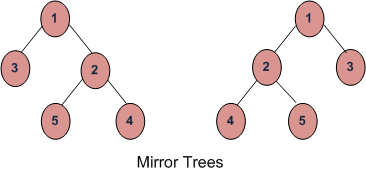Skip to content
Related Articles
Check if two trees are Mirror
• Difficulty Level : Easy
• Last Updated : 10 May, 2021

Given two Binary Trees, write a function that returns true if two trees are mirror of each other, else false. For example, the function should return true for following input trees.This problem is different from the problem discussed here.
For two trees ‘a’ and ‘b’ to be mirror images, the following three conditions must be true:

1. Their root node’s key must be same
2. Left subtree of root of ‘a’ and right subtree root of ‘b’ are mirror.
3. Right subtree of ‘a’ and left subtree of ‘b’ are mirror.

Below is implementation of above idea.

## C++

 `// C++ program to check if two trees are mirror``// of each other``#include``using` `namespace` `std;` `/* A binary tree node has data, pointer to``   ``left child and a pointer to right child */``struct` `Node``{``    ``int` `data;``    ``Node* left, *right;``};` `/* Given two trees, return true if they are``   ``mirror of each other */``int` `areMirror(Node* a, Node* b)``{``    ``/* Base case : Both empty */``    ``if` `(a==NULL && b==NULL)``        ``return` `true``;` `    ``// If only one is empty``    ``if` `(a==NULL || b == NULL)``        ``return` `false``;` `    ``/* Both non-empty, compare them recursively``     ``Note that in recursive calls, we pass left``     ``of one tree and right of other tree */``    ``return`  `a->data == b->data &&``            ``areMirror(a->left, b->right) &&``            ``areMirror(a->right, b->left);``}` `/* Helper function that allocates a new node */``Node* newNode(``int` `data)``{``    ``Node* node = ``new` `Node;``    ``node->data  = data;``    ``node->left  =  node->right  = NULL;``    ``return``(node);``}` `/* Driver program to test areMirror() */``int` `main()``{``    ``Node *a = newNode(1);``    ``Node *b = newNode(1);``    ``a->left = newNode(2);``    ``a->right = newNode(3);``    ``a->left->left  = newNode(4);``    ``a->left->right = newNode(5);` `    ``b->left = newNode(3);``    ``b->right = newNode(2);``    ``b->right->left = newNode(5);``    ``b->right->right = newNode(4);` `    ``areMirror(a, b)? cout << ``"Yes"` `: cout << ``"No"``;` `    ``return` `0;``}`

## Java

 `// Java program to see if two trees``// are mirror of each other` `// A binary tree node``class` `Node``{``    ``int` `data;``    ``Node left, right;` `    ``public` `Node(``int` `data)``    ``{``        ``this``.data = data;``        ``left = right = ``null``;``    ``}``}` `class` `BinaryTree``{``    ``Node a, b;``    ` `    ``/* Given two trees, return true if they are``       ``mirror of each other */``    ``boolean` `areMirror(Node a, Node b)``    ``{``        ``/* Base case : Both empty */``        ``if` `(a == ``null` `&& b == ``null``)``            ``return` `true``;` `        ``// If only one is empty``        ``if` `(a == ``null` `|| b == ``null``)``            ``return` `false``;` `        ``/* Both non-empty, compare them recursively``           ``Note that in recursive calls, we pass left``           ``of one tree and right of other tree */``        ``return` `a.data == b.data``                ``&& areMirror(a.left, b.right)``                ``&& areMirror(a.right, b.left);``    ``}` `    ``// Driver code to test above methods``    ``public` `static` `void` `main(String[] args)``    ``{``        ``BinaryTree tree = ``new` `BinaryTree();``        ``Node a = ``new` `Node(``1``);``        ``Node b = ``new` `Node(``1``);``        ``a.left = ``new` `Node(``2``);``        ``a.right = ``new` `Node(``3``);``        ``a.left.left = ``new` `Node(``4``);``        ``a.left.right = ``new` `Node(``5``);` `        ``b.left = ``new` `Node(``3``);``        ``b.right = ``new` `Node(``2``);``        ``b.right.left = ``new` `Node(``5``);``        ``b.right.right = ``new` `Node(``4``);` `        ``if` `(tree.areMirror(a, b) == ``true``)``            ``System.out.println(``"Yes"``);``        ``else``            ``System.out.println(``"No"``);` `    ``}``}` `// This code has been contributed by Mayank Jaiswal(mayank_24)`

## Python3

 `# Python3 program to check if two``# trees are mirror of each other` `# A binary tree node``class` `Node:``    ``def` `__init__(``self``, data):``        ``self``.data ``=` `data``        ``self``.left ``=` `None``        ``self``.right ``=` `None` `# Given two trees, return true``# if they are mirror of each other``def` `areMirror(a, b):``    ` `    ``# Base case : Both empty``    ``if` `a ``is` `None` `and` `b ``is` `None``:``        ``return` `True``    ` `    ``# If only one is empty``    ``if` `a ``is` `None` `or` `b ``is` `None``:``        ``return` `False``    ` `    ``# Both non-empty, compare them``    ``# recursively. Note that in``    ``# recursive calls, we pass left``    ``# of one tree and right of other tree``    ``return` `(a.data ``=``=` `b.data ``and``            ``areMirror(a.left, b.right) ``and``            ``areMirror(a.right , b.left))` `# Driver code``root1 ``=` `Node(``1``)``root2 ``=` `Node(``1``)` `root1.left ``=` `Node(``2``)``root1.right ``=` `Node(``3``)``root1.left.left ``=` `Node(``4``)``root1.left.right ``=` `Node(``5``)` `root2.left ``=` `Node(``3``)``root2.right ``=` `Node(``2``)``root2.right.left ``=` `Node(``5``)``root2.right.right ``=` `Node(``4``)` `if` `areMirror(root1, root2):``    ``print` `(``"Yes"``)``else``:``    ``print` `(``"No"``)` `# This code is contributed by AshishR`

## C#

 `using` `System;` `// c# program to see if two trees``// are mirror of each other` `// A binary tree node``public` `class` `Node``{``    ``public` `int` `data;``    ``public` `Node left, right;` `    ``public` `Node(``int` `data)``    ``{``        ``this``.data = data;``        ``left = right = ``null``;``    ``}``}` `public` `class` `BinaryTree``{``    ``public` `Node a, b;` `    ``/* Given two trees, return true if they are``    ``mirror of each other */``    ``public` `virtual` `bool` `areMirror(Node a, Node b)``    ``{``        ``/* Base case : Both empty */``        ``if` `(a == ``null` `&& b == ``null``)``        ``{``            ``return` `true``;``        ``}` `        ``// If only one is empty``        ``if` `(a == ``null` `|| b == ``null``)``        ``{``            ``return` `false``;``        ``}` `        ``/* Both non-empty, compare them recursively``        ``Note that in recursive calls, we pass left``        ``of one tree and right of other tree */``        ``return` `a.data == b.data && areMirror(a.left, b.right)``                            ``&& areMirror(a.right, b.left);``    ``}` `    ``// Driver code to test above methods``    ``public` `static` `void` `Main(``string``[] args)``    ``{``        ``BinaryTree tree = ``new` `BinaryTree();``        ``Node a = ``new` `Node(1);``        ``Node b = ``new` `Node(1);``        ``a.left = ``new` `Node(2);``        ``a.right = ``new` `Node(3);``        ``a.left.left = ``new` `Node(4);``        ``a.left.right = ``new` `Node(5);` `        ``b.left = ``new` `Node(3);``        ``b.right = ``new` `Node(2);``        ``b.right.left = ``new` `Node(5);``        ``b.right.right = ``new` `Node(4);` `        ``if` `(tree.areMirror(a, b) == ``true``)``        ``{``            ``Console.WriteLine(``"Yes"``);``        ``}``        ``else``        ``{``            ``Console.WriteLine(``"No"``);``        ``}` `    ``}``}` `// This code is contributed by Shrikant13`

## Javascript

 ``

Output :

`Yes`

Time Complexity : O(n)
Iterative method to check if two trees are mirror of each other

?list=PLqM7alHXFySHCXD7r1J0ky9Zg_GBB1dbk
This article is contributed by Ashish Gupta. Please write comments if you find anything incorrect, or you want to share more information about the topic discussed above

Attention reader! Don’t stop learning now. Get hold of all the important DSA concepts with the DSA Self Paced Course at a student-friendly price and become industry ready.  To complete your preparation from learning a language to DS Algo and many more,  please refer Complete Interview Preparation Course.

In case you wish to attend live classes with industry experts, please refer DSA Live Classes

My Personal Notes arrow_drop_up